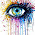## Thursday, July 10, 2014

### Time & Work Basic Question Solving - 11 July 2014

1. X is twice good a workman as Y and together they finish a piece of work in 18 days. In how many days will X alone finish the work ?

Solution - According to the condition given if X is twice good a workman as Y then the time taken by X to finish a particular work would be half of the time taken by Y to finish the same work.

So if we suppose that Y takes 2k days to finish a work then X would take k days to finish the same work.

Solving,  1/(2k) + 1/(k) = 1/18

k = 27 days

Answer - X would finish the work in 27 days.

2. If 10 men complete a work in 12 days. How many days would be taken by 18 men to complete the same work ?

Solution - Suppose the time taken by 18 men to complete the same work is t days.

Using the concept of Mandays -

10*12 = 18*t

t = 20/3 days

Answer - 18 men would take 20/3 days to complete the same work.

3. If 100 men can do 100 jobs in 100 days, 1 men can do one job in how many days ?

Solution - Using the concept M*D = W

(100*100)/(1*D) = 100/1

Solving we get D = 100 days

Answer - 1 men can do one job in 100 days.

4. 1 man or 2 boys or 3 girls can do a piece of work in 121 days. In how many days can one man, one girl and one boy do the same work ?

Solution - Let one man does m units of work in 1 day.

Let one boy does b units of work in 1 day.

Let one girl does g units of work in 1 day.

So 1m*121 = 2b*121 = 3g*121 = W, where W is the total units of work.

m = W/121 , b= W/242 , g = W/ 363

So 1 man, 1 girl and 1 boy can do the same work in   (W) / ((W/121) + (W/242) + (W/363)) = 66

Answer - So 1 man, 1 girl and 1 boy can do the same work in 66 days.

5. If the ratio of work efficiency of P & Q is 6:5 and that of Q & R is 6:5. If P can complete the piece of work in 2 days, In how many days the same work can be completed by Q & R separately ?

Solution - If the work efficiency of P & Q is 6:5 then time taken would be in the ratio 5:6

If the work efficiency of Q & R is 6:5 then time taken would be in the ratio 5:6

Let the time taken by P = 5K and by Q = 6K, where K is some constant.

5K = 2, So 6K = 12/5 Days

So the time taken by Q to complete the work is 12/5 days

Now let the time taken by Q = 5X and by R = 6X, where X is some constant.

Q = 12/5 days, So R = 72/25 days

Answer - So the time taken by R to complete the work is 72/25 days.

1.Can u plz explain. Soln of question 3

2.Its (M1*H1*D1)/W1 = (M2*H2*D2)/W2 just substitute value M=men H =hours D=days W=work accordingly

1.This comment has been removed by a blog administrator.

2.This comment has been removed by a blog administrator.

3.nice solution.

Add a Comment or Query